# 1 C++简介

## 1.1 起源

-贝尔实验室20世纪80年代(1979)

• 文字处理程序以及电子表格
• 编译器
• 操作系统
• 大型游戏等

## 1.3 C++和C

• C语言是结构化和模块化的语言，面向过程。
• C++保留了C语言原有的所有优点，增加了面向对象的机制，俗称“带类的C",1983年更名为C++

# 2开发工具

• Visual C++ 6.0：经典开发工具，与流行操作系统有冲突
• VS 2015等：功能强大，体积也强大
• Code::Blocks：开源免费开发工具，专业开发人员推荐使用
• 其他开发工具：DeV C++、CLion、C-Free、Xcode、C4droid

# 3 基本语法

• 对象-对象具有状态的行为。对象是类的实例。
• 类-类可以定义为对象行为、状态的模版。
• 方法-从基本上讲，一个方法表示一种行为，一个类可以包含多种方法。
• 变量

### 3.1 注释

//单行注释
/*

*/


### 3.2关键字

asmelsenewthis
autoenumoperatorthrow
boolexplicitprivatetrue
breakexportprotectedtry
caseexternpublictypedef
catchfalseregistertypeid
charfloatreinterpret_casttypename
classforreturnunion
constfriendshortunsigned
const_castgotosignedusing
continueifsizeofvirtual
defaultinlinestaticvoid
deleteintstatic_castvolatile
dolongstructwchar_t
doublemutableswitchwhile
dynamic_castnamespacetemplate

### 3.3标识符

• 标识符是用来标识变量、函数、类、模块，或任何其他用户自定义项目的名称。一个标识符以字母 A-Z 或 a-z 或下划线 _ 开始，后跟零个或多个字母、下划线和数字（0-9）。
• 标识符内不允许出现标点字符，比如 @、& 和 %。C++ 是区分大小写的编程语言

# 4 数据类型

### 4.1基本数据类型

void0
bool1{true.false}
wchar_t2或4个字节
char(signed char)1-128~+127
short(signed short)2-32768~+32767
int(signed int)4-2147483648~+2147483647
long(signed long)4-2147483648~+2147483647
long long(signed long long)8-9,223,372,036,854,775,808 ~9,223,372,036,854,775,807
float4-.34*1038~3.4*1038
double8-1.7*10308~1.7*10308
unsigned char10~255
unsigned shrot20~65525
unsigned(unsigned int)40~4294967295
unsigned long40~4294967295
unsigned long long80 ~ 18,446,744,073,709,551,615
//x64处理器 64位window10 vs2015
#include <iostream>
using namespace std;
int main()
{
bool b;
char c;short s; int i; long l; long long ll; float f; double d; long double ld;long float lf;
unsigned char uc; unsigned short us; unsigned int ui; unsigned long ul; unsigned long long ull;
cout << sizeof(bool) <<  endl;
cout << sizeof(char)<<" " << sizeof(short)<<" "<< sizeof(signed int) << " " << sizeof(long) << " " << sizeof(signed long long) << " " << sizeof(float) << " " << sizeof(double) << " " << sizeof(long float) << " " << sizeof(long double) << endl;
cout <<sizeof(unsigned char)<<" "<< sizeof(unsigned short) << " " << sizeof(unsigned int) << " " << sizeof(unsigned long) << " " << sizeof(unsigned long long) << endl;
cout << sizeof(unsigned) << endl;

cout << "hello World!!!" <<endl;
system("pause");
return 0;
}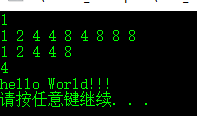### 4.2 数据类型在不同系统中所占空间大小

这个与机器、操作系统、编译器有关。比如同样是在32bits的操作系统系，VC++的编译器下int类型为占4个字节；而tuborC下则是2个字节。

• c/c++规定int字长和机器字长相同
• 操作系统字长和机器字长未必一致
• 编译器根据操作系统字长来定义int字长

char111
char*248
short222
int244
long448
long long888

### 4.3 typedef声明

//使用typedef为一个已有的类型取一个新的名字,语法如下：
typedef type newname
//eg：
typedef int feet
feet distance


### 4.4 枚举类型

C++中的一种派生数据类型，它是由用户定义的若干枚举常量的集合；枚举元素是一个整型，枚举型可以隐式的转换为int型，int型不能隐式的转换为枚举型。

//枚举类型的语法:
enum 枚举名{
标识符[=整型常数],
标识符[=整型常数],
...
标识符[=整型常数]
}枚举变量;


• 默认情况下，第一个名称的值为 0，第二个名称的值为 1，第三个名称的值为 2，以此类推。但是，您也可以给名称赋予一个特殊的值，只需要添加一个初始值即可。
例如：
enum course {math,chinese,english,physics,chemistry}c;
c = english;
cout<<c<<endl;  //2
//english为1 physics为2 chemistry为3，chinese仍为1，math仍为0
enum course {math,chinese,english=1,physics,chemistry};


# 5 变量

### 5.1 变量的声明和定义

• 变量声明向编译器保证变量以给定的类型和名称存在，这样编译器在不需要知道变量完整细节的情况下也能继续进一步的编译。
• 可以在 C++ 程序中多次声明一个变量，但变量只能在某个文件、函数或代码块中被定义一次。
• 多个变量赋同一个值时，需要分别赋值。
int x = y = z = 66;//错误
int x = 3,y = 3,z = 3;
int x, y ,z = 3;
x = y = z;


// 变量声明
extern int a, b;
int main ()
{
// 变量定义
int a, b;
// 初始化
a = 23;
b = 25;
return 0;
}


### 5.2 变量的作用域

• 局部变量和全局变量的名称可以相同，但是在函数内，局部变量的值会覆盖全局变量的值。
• 当局部变量被定义时，系统不会对其初始化；定义全局变量时，系统会自动初始化值：int float double 0，char ’\0‘，指针 NULL
int i = 66;
int main ()
{
int i = 88;
cout << i<<endl;//8
return 0;
}
float f;
double d;
char c;
int *p;
int main()
{
cout << i << f << d << c << p << endl;//000 00000000
return 0
}


# 6 运算符

• 算术运算符：+ - * / % ++ --
• 关系运算符：== != < > >= <=
• 逻辑运算符：&& || !
• 位运算符：& | ^ ~ << >>
• 赋值运算符：= += -= *= /= %= <<= >>= &= ^= !=
• 杂项运算符：
sizeof            //返回变量的大小，eg:sizeof(a)返回4 a是整型  sizeof(int)
Condition?X:Y     //三元运算符 Condition为true，值为X，否则值为Y
,                //逗号表达式，值为最后一个表达式的值
.和->            //用于引用类、结构和公用体的成员
Cast            //强制类型转换符  eg:int(2.202)返回2
&              //指针运算符 返回变量的地址
*             //指针运算符  指向一个变量

• 运算符优先级

# 7 语法结构

### 7.1 循环结构

• while
while(conditon)//0为false,非0为true
{
statement(s);
}

• for
for(init;conditon;increment)//0为false,非0或什么也不写为true
{
statement(s);
}


1.init首先被执，且只会执行一次，也可以不写任何语句。
2.然后会判断conditon,true执行循环主体，false跳过循环
3.执行完循环主体，执行increment,跳到2

int array = { 11, 22, 33, 44, 55 };
for (int x : array)
{
cout << x << " ";
}
cout << endl;
// auto 类型也是 C++11 新标准中的，用来自动获取变量的类型
for (auto x : array)
{
cout << x << " ";
}

• for each
STL中的for增强循环。
int a = { 4,3,2,1 };
for each (int var in a)
{
cout << var << " ";
}


### 7.2 判断结构

• if
if(expr)
{
statement;//如果expr为true将执行的语句块
}
if(expr)
{
statement1;// 如果expr为true将执行的语句块
}
else
{
statement2;// 如果expr为false将执行的语句
}
if(expr1)
{
statement1;// 如果expr1为true将执行的语句块
}
elseif(expr2)
{
statement2;// 如果expr2为true将执行的语句块
}
...
else
{
statementElse;// 当上面的表达式都为false执行的语句块
}

• switch
switch(expression){
case constant-expression  :
statement(s);
break;
case constant-expression  :
statement(s);
break;
// 您可以有任意数量的 case 语句
default : // 可选的
statement(s);
}

• 每个case后满的常量表达式必须各不相同。
• case语句和default语句出现的顺序对执行结果没有影响。
• 若case后没有break,执行完就不会判断，继续执行下一个case语句。直到遇到brerak。
• default后面如果没有case，则break可以省略
• 多个case可以用一组执行语句
char c = 'A';
switch (c)
{

case 'A':
case 'B':
case 'C':
cout << "及格了" << endl;
break;
default:
cout << "不及格" << endl;

}


### 7.3 三元运算符

//如果 Exp1 为真，则计算 Exp2 的值，结果即为整个 ? 表达式的值。如果 Exp1 为假，则计算 Exp3 的值，结果即为整个 ? 表达式的值
Exp1 ? Exp2 : Exp3;


### 7.4 预处理命令

• 宏定义：#define 标识符 字符串
• 文件包含：#include<filename> 或者#include“filename”
• 条件编译
//如果标识符被#define定义过，执行程序段1，否则执行程序段2
#ifdef 标识符
程序段1
#else
程序段2
#endif
//如果标识符没有被#define定义过，执行程序段1，否则执行程序段2
#ifndef 标识符
程序段1
#else
程序段2
#endif
//如果表达式为true，执行程序段1，否则执行程序段2
#if 表达式
程序段1
#else
程序段2
#endif


# 8 数组

## 8.1一维数组

• 初始化数组时，可以只给部分数组元素赋值
• 对全部元素数组赋值时，可以不指定数组长度，编译系统会根据初值个数确定数组的长度。
• static型数组元素不赋初值，系统会自动默认为0。
int arr1 = {1,2,3,4};
int arr2 = { 1,2 };
int arr = {0];//所有元素为0
static int arr3;
int arr4;
cout << "arr1:"<<arr1 << arr1 << arr1 << arr1 << endl;
cout << "arr2:" << arr2 << arr2 << arr2 << arr2 << endl;
cout << "arr3:" << arr3 << arr3 << arr3 << arr3 << endl;
cout << "arr4:" << arr4 << arr4 << arr4 << arr4 << endl;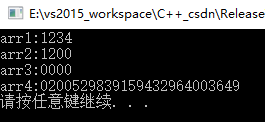## 8.2二维数组

• 将所有数据写在一个花括号内，自动按照数组元素个数在内存中排列的顺序赋值
• 可对部分元素赋值，其余元素的值自动取0.
• 定义初始化数组时，可以省略第一维的长度，第二维不能省，系统会自动确认行数
int arr1;
int arr = {0];//所有元素为0
int arr2 = { {1,2,3},{4,5,6} };
int arr3 = { 1,2,3 ,4,5,6 };
int arr4 = { {1},{4,6} };
int arr5[] = { 1,2,3 ,4,5,6 };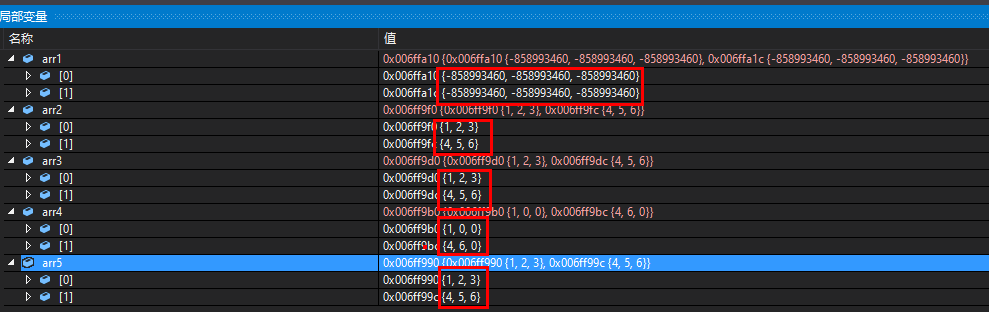char类型的数组，在字符数组中最后一位为’\0’）时，可以看成时字符串。在C++中定义了string类，在Visual C++中定义了Cstring类。

//字符串长度为8个字节,最后一位是'\0'。
char array = "yuanrui";//yuanrui\0\0\0
//也可以不用定义字符串长度，如：
char arr[] = "yuanrui";//yuanrui\0


## 8.3 指向数组的指针

double *p;
double arr;
p = arr;//p = &arr;
*(p+3);//arr


## 8.4 数组与new(动态创建数组)

int* arr1 = new int;//delete []arr1;
int* arr2 = new int{ 1,2 };//delete []arr2


int m=2, n=3;
int** arr3 = new int*;//delete []arr3
for (int i = 0; i < 10; ++i)
{
arr3[i] = new int; // delete []arr3[i]
}
int* arr4 = new int[m*n];//数据按行存储 delete []arr3


## 8.5 数组与函数

### 数组->函数

• 如果传递二维数组，形参必须制定第二维的长度。
形式参数是一个指针：void function(int *param)
形式参数是一个已定义大小的数组：void function(int param)
形式参数是一个未定义大小的数组：void function(int param[])
二维数组：void function(int a[],int size)

### 函数返回数组

• C++ 不支持在函数外返回局部变量的地址，除非定义局部变量为 static 变量。
int * function();
int** function();


## 8.6 获取数组的大小

• 动态创建(new)的基本数据类型数组无法取得数组大小
int a;
//第一种方法
cout<<sizeof(a)/sizeof(a)<<endl;
//第二种方法
cout << end(a) - begin(a) << endl;
//二维数组
int arr;
int lines = sizeof(arr) / sizeof(arr);
int row = sizeof(arr) / sizeof(arr);//行
int col = lines / row;//列
cout << row << "::"<<col << endl;
cout << end(arr) - begin(arr) << endl;//5行


# 9 函数

函数是实现模块化程序设计思想的重要工具， C++程序中每一项操作基本都是由一个函数来实现的，C++程序中只能有一个主函数（main）

### 9.1 函数声明与定义

• 函数类型-函数的返回值类型；函数名-必须符合C++标识符命名规则，后面必须跟一对括号；函数体-实现函数功能的主题部分；参数列表-函数名后面的括号内，用于向函数传递数值或带回数值。
• 函数声明中，参数名可以省略，参数类型和函数的类型不能省略。
• 函数声明可以放在主调函数内部，放在调用语句之前；也可以放在主调函数外，如果位于所有定义函数之前，后面函数定义顺序任意，各个主调函数调用也不必再做声明
• 当函数定义在前，函数调用灾后，可以不用函数声明。
后两条总结一下就是：调用函数前，程序得知道有这个函数，声明就是提前让程序知道有这么的玩意
函数声明：
函数类型 函数名(参数列表);
eg:
int max(int a,int b);//声明函数时，a,b可以省略
int max(int,int);
void show();


函数类型 函数名(参数列表)
{
函数体;
}
eg:
int max(int a,int b)
{
int z;
z = a>b?a:b;
return z;
}


### 9.2 函数的参数与返回值

• 形参：函数定义后面括号里的参数，函数调用前不占内存。
• 实参：函数调用括号里的参数，可以是常量，变量或表达式等。
形参和实参必须个数相同、类型一致，顺序一致
函数传递方式：传值，指针，引用
关于指针和引用后面有详细介绍。
//传值-修改函数内的形式参数对实际参数没有影响
{
value++;
return value;
}
int main()
{
int v = 10;
cout << "v = " << v << endl;//v = 10
return 0;
}
//指针-修改形式参数会影响实际参数
{
(*pValue)++;
return *pValue;
}
int main()
{
int v = 10;
cout << "v = " << v << endl;//v = 11
return 0;
}
//引用-修改形式参数会影响实际参数
{
value++;
return value;
}
int main()
{
int v = 10;
cout << "v = " << v << endl;//v = 11
return 0;
}


int sum(int a, int b=2)
{
return (a + b);
}

int main ()
{
cout << "Total value is :" << sum(100, 200);<< endl;//Total value is :300
cout << "Total value is :" << sum(100);<< endl;//Total value is :102
return 0;
}


• 返回值通过return给出，return后面跟表达式，且只能放回一个值;如果没有表达式，可以不写return；return后面的括号可有可无。
• return语句中的表达式类型应与函数类型一致，否则自动转换类型（函数类型决定返回值类型）

### 9.3 函数调用

• 函数可以单独作为一个语句使用。有返回值的函数，可将函数调用作为语句的一部分，利用返回值参与运算。
函数调用形式：参数传递–>函数体执行–>返回主调函数
函数名(实参列表);
show();


int a()
{
return 666;
}
int b(int sum)
{
return sum+a()
}
int main()
{
cout<<b(222)<<endl;//888
return 0;
}


• 一个函数直接或间接递归调用该函数本身，称为函数的递归调用
• 递归和回归：原问题=>子问题 子问题的解=>原问题的解
//直接递归调用：求1+...n的值
int total(int sum)
{
if (sum == 1)
{
return 1;
}
return sum + total(sum - 1);
}
int main()
{
cout << "total = " << total(10) << endl;//total = 55
system("pause");
return 0;
}
//间接递归调用
int f2();
int f1()
{
...
f2()
}
int f2()
{
f1();
}


### 9.4 函数重载

同一个函数名对应不同的函数实现，每一类实现对应着一个函数体，名字相同，功能相同，只是参数的类型或参数的个数不同。

int add(int a,int b)
{
return a+b;
}
{
return a+b;
}
{
return a+b+c;
}


### 9.5 内联(inline)函数

c++在编译时可以讲调用的函数代码嵌入到主调函数中，这种嵌入到主调函数中的函数称为内联函数，又称为内嵌函数或内置函数。

• 定义内联函数时，在函数定义和函数原型声明时都使用inline，也可以只在其中一处使用，其效果一样。
• 内联函数在编译时用内联函数函数的函数体替换,所以不发生函数调用，不需要保护现场，恢复现场，节省了开销。
• 内联函数增加了目标程序的代码量。因此，一般只将函数规模很小且使用频繁的函数声明为内联函数。
• 当内联函数中实现过于复杂时，编译器会将它作为一个普通函数处理,所以内联函数内不能包含循环语句和switch语句。
内联函数格式如下：
inline 函数类型 函数名(形参列表)
{
函数体;
}
inline int add(int a, int b)
{
return a + b;
}


### 9.6 洞悉内联函数底层原理

1.使用Visual Studio 2015创建一个C++Win32控制台程序,点击项目->项目属性设置内联函数优化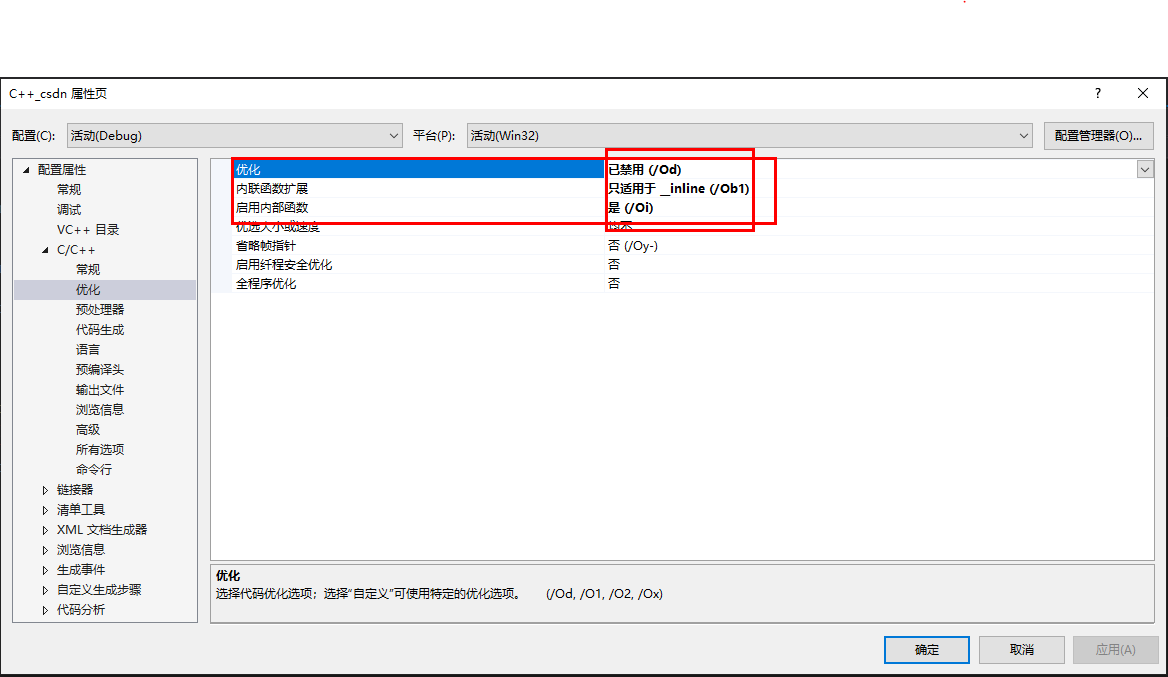2.编写内联函数代码，设置断点，debug启动

#include <iostream>
#include <string>
using namespace std;
inline int add(int a, int b)
{
return a + b;//断点1
}
int main()
{
cout << result << endl;//断点2
return 0;
}


3.调试->窗口->反汇编，然后就能看到编译后的汇编程序

...
00B620DE  mov         eax,0Ch
00B620E6  mov         dword ptr [result],eax
cout << result << endl;
00B620E9  mov         esi,esp
00B620EB  push        offset std::endl<char,std::char_traits<char> > (0B610A5h)
00B620F0  mov         edi,esp
00B620F2  mov         eax,dword ptr [result]
00B620F5  push        eax
00B620F6  mov         ecx,dword ptr [_imp_?cout@std@@3V?$basic_ostream@DU?$char_traits@D@std@@@1@A (0B6D098h)]
00B620FC  call        dword ptr [__imp_std::basic_ostream<char,std::char_traits<char> >::operator<< (0B6D0A8h)]
00B62102  cmp         edi,esp
00B62104  call        __RTC_CheckEsp (0B611C7h)
00B62109  mov         ecx,eax
00B6210B  call        dword ptr [__imp_std::basic_ostream<char,std::char_traits<char> >::operator<< (0B6D0ACh)]
00B62111  cmp         esi,esp
00B62113  call        __RTC_CheckEsp (0B611C7h)
return 0;


4.从汇编代码中可以代码编译后内联函数直接嵌入到主函数中，并且断点1不会执行到，下面是没使用内联函数(去掉inline关键字)的汇编代码:

int result = add(12, 34);
00291A4E  push        22h
00291A50  push        0Ch
00291A5A  mov         dword ptr [result],eax
cout << result << endl;
00291A5D  mov         esi,esp
00291A5F  push        offset std::endl<char,std::char_traits<char> > (02910A5h)
00291A64  mov         edi,esp
00291A66  mov         eax,dword ptr [result]
00291A69  push        eax
00291A6A  mov         ecx,dword ptr [_imp_?cout@std@@3V?$basic_ostream@DU?$char_traits@D@std@@@1@A (029D098h)]
cout << result << endl;
00291A70  call        dword ptr [__imp_std::basic_ostream<char,std::char_traits<char> >::operator<< (029D0A8h)]
00291A76  cmp         edi,esp
00291A78  call        __RTC_CheckEsp (02911C7h)
00291A7D  mov         ecx,eax
00291A7F  call        dword ptr [__imp_std::basic_ostream<char,std::char_traits<char> >::operator<< (029D0ACh)]
00291A85  cmp         esi,esp
00291A87  call        __RTC_CheckEsp (02911C7h)
system("pause");
00291A8C  mov         esi,esp
00291A8E  push        offset string "pause" (0299B30h)
00291A93  call        dword ptr [__imp__system (029D1DCh)]
00291A9C  cmp         esi,esp
00291A9E  call        __RTC_CheckEsp (02911C7h)
return 0;


5.在内联函数中添加几个循环后，编译器就把内联函数当做普通函数看待了，代码如下：

inline int add(int a, int b)
{

int sum = 0;
for (int i = 0; i < 100; i++)
a++;
for (int i = 0; i < 100; i++)
{

for (int i = 0; i < 100; i++)
{
sum++;
}
}

return a + b;
}
int main()
{
cout << result << endl;
return 0;
}

	int result = add(12, 34);
00181A4E  push        22h
00181A50  push        0Ch
00181A5A  mov         dword ptr [result],eax
cout << result << endl;
00181A5D  mov         esi,esp
00181A5F  push        offset std::endl<char,std::char_traits<char> > (01810A5h)
00181A64  mov         edi,esp
00181A66  mov         eax,dword ptr [result]
00181A69  push        eax
00181A6A  mov         ecx,dword ptr [_imp_?cout@std@@3V?$basic_ostream@DU?$char_traits@D@std@@@1@A (018D098h)]
cout << result << endl;
00181A70  call        dword ptr [__imp_std::basic_ostream<char,std::char_traits<char> >::operator<< (018D0A8h)]
00181A76  cmp         edi,esp
00181A78  call        __RTC_CheckEsp (01811C7h)
00181A7D  mov         ecx,eax
00181A7F  call        dword ptr [__imp_std::basic_ostream<char,std::char_traits<char> >::operator<< (018D0ACh)]
00181A85  cmp         esi,esp
00181A87  call        __RTC_CheckEsp (01811C7h)
return 0;
00181AA3  xor         eax,eax


# 10 字符串(string)

### 10.1 C风格的字符串(字符数组)

C风格的字符串实际上是使用 null 字符 ‘\0’ 终止的一维字符数组。

• 输入字符串长度一定小于已定义的字符数组长度，最后一位是/0终止符号；不然输出时无法知道在哪里结束。
字符数组的定义和初始化
char a
//字符个数不够，补0; 字符个数超过报错
char str = {'h','e','i','r','e','n'};
char str[] = {'h','e','i','r','e','n'};
cin>>str;//输入 输入字符串长度一定小于已定义的字符数组长度
cout<<str;//输出


strcat(char s1[],const char s2[]);//将s2接到s1上
strcpy(char s1[],const char s2[]);//将s2复制到s1上
strcmp(const char s1[],const char s2[]);//比较s1,s2 s1>s2返回1 相等返回1，否则返回-1
strlen(char s[]);//计算字符串s的长度 字符串s的实际长度，不包括\0在内


### 10.2 C++中的字符串(string)

//定义
string 变量;
string str1;
//赋值
string str2 = "ShangHai";
string str3 = str2;
str3 = '2';//对某个字符赋值
//字符串数组
string 数组名[常量表达式]
string arr;


#include <iostream>
#include <algorithm>
#include <string>
string str;//生成空字符串
string s(str);//生成字符串为str的复制品
string s(str, strbegin,strlen);//将字符串str中从下标strbegin开始、长度为strlen的部分作为字符串初值
string s(cstr, char_len);//以C_string类型cstr的前char_len个字符串作为字符串s的初值
string s(num ,c);//生成num个c字符的字符串
string s(str, stridx);//将字符串str中从下标stridx开始到字符串结束的位置作为字符串初值
size()和length();//返回string对象的字符个数
max_size();//返回string对象最多包含的字符数，超出会抛出length_error异常
capacity();//重新分配内存之前，string对象能包含的最大字符数
>,>=,<,<=,==,!=//支持string与C-string的比较(如 str<”hello”)。  使用>,>=,<,<=这些操作符的时候是根据“当前字符特性”将字符按字典顺序进行逐一得 比较，string (“aaaa”) <string(aaaaa)。
compare();//支持多参数处理，支持用索引值和长度定位子串来进行比较。返回一个整数来表示比较结果，返回值意义如下：0：相等 1：大于 -1：

push_back()
insert( size_type index, size_type count, CharT ch );//在index位置插入count个字符ch
insert( size_type index, const CharT* s );//index位置插入一个常量字符串
insert( size_type index, const CharT* s, size_type n);//index位置插入常量字符串
insert( size_type index, const basic_string& str );//index位置插入常量string中的n个字符
insert( size_type index, const basic_string& str, size_type index_str, size_type n);//index位置插入常量str的从index_str开始的n个字符
insert( size_type index, const basic_string& str,size_type index_str, size_type count = npos);//index位置插入常量str从index_str开始的count个字符，count可以表示的最大值为npos.这个函数不构成重载 npos表示一个常数，表示size_t的最大值，string的find函数如果未找到指定字符，返回的就是一个npos
iterator insert( iterator pos, CharT ch );
iterator insert( const_iterator pos, CharT ch );
void insert( iterator pos, size_type n, CharT ch );//迭代器指向的pos位置插入n个字符ch
iterator insert( const_iterator pos, size_type count, CharT ch );//迭代器指向的pos位置插入count个字符ch
void insert( iterator pos, InputIt first, InputIt last );
iterator insert( const_iterator pos, InputIt first, InputIt last );
append() 和 + 操作符
//访问string每个字符串
string s1("yuanrui"); // 调用一次构造函数
// 方法一： 下标法
for( int i = 0; i < s1.size() ; i++ )
cout<<s1[i];
// 方法二：正向迭代器
for( string::iterator iter = s1.begin();; iter < s1.end() ; iter++)
cout<<*iter;
// 方法三：反向迭代器
for(string::reverse_iterator riter = s1.rbegin(); ; riter < s1.rend() ; riter++)
cout<<*riter;
iterator erase(iterator p);//删除字符串中p所指的字符
iterator erase(iterator first, iterator last);//删除字符串中迭代器区间[first,last)上所有字符
string& erase(size_t pos = 0, size_t len = npos);//删除字符串中从索引位置pos开始的len个字符
void clear();//删除字符串中所有字符
string& replace(size_t pos, size_t n, const char *s);//将当前字符串从pos索引开始的n个字符，替换成字符串s
string& replace(size_t pos, size_t n, size_t n1, char c); //将当前字符串从pos索引开始的n个字符，替换成n1个字符c
string& replace(iterator i1, iterator i2, const char* s);//将当前字符串[i1,i2)区间中的字符串替换为字符串s
//tolower()和toupper()函数 或者 STL中的transform算法
string s = "ABCDEFG";
for( int i = 0; i < s.size(); i++ )
s[i] = tolower(s[i]);
transform(s.begin(),s.end(),s.begin(),::tolower);
size_t find (constchar* s, size_t pos = 0) const;//在当前字符串的pos索引位置开始，查找子串s，返回找到的位置索引，-1表示查找不到子串
size_t find (charc, size_t pos = 0) const;//在当前字符串的pos索引位置开始，查找字符c，返回找到的位置索引，-1表示查找不到字符
size_t rfind (constchar* s, size_t pos = npos) const;//在当前字符串的pos索引位置开始，反向查找子串s，返回找到的位置索引，-1表示查找不到子串
size_t rfind (charc, size_t pos = npos) const;//在当前字符串的pos索引位置开始，反向查找字符c，返回找到的位置索引，-1表示查找不到字符
size_tfind_first_of (const char* s, size_t pos = 0) const;//在当前字符串的pos索引位置开始，查找子串s的字符，返回找到的位置索引，-1表示查找不到字符
size_tfind_first_not_of (const char* s, size_t pos = 0) const;//在当前字符串的pos索引位置开始，查找第一个不位于子串s的字符，返回找到的位置索引，-1表示查找不到字符
size_t find_last_of(const char* s, size_t pos = npos) const;//在当前字符串的pos索引位置开始，查找最后一个位于子串s的字符，返回找到的位置索引，-1表示查找不到字符
size_tfind_last_not_of (const char* s, size_t pos = npos) const;//在当前字符串的pos索引位置开始，查找最后一个不位于子串s的字符，返回找到的位置索引，-1表示查找不到子串
sort(s.begin(),s.end());
substr(pos,n);//返回字符串从下标pos开始n个字符
strtok()
char str[] = "I,am,a,student; hello world!";
const char *split = ",; !";
char *p2 = strtok(str,split);
while( p2 != NULL )
{
cout<<p2<<endl;
p2 = strtok(NULL,split);
}


# 11 指针和引用

### 11.1 指针

• 数据类型是指针变量所指向的变量的数据类型，*表示其后的变量为指针变量
数据类型 *指针变量名;
int    *ip;    //整型的指针
double *dp;    //double 型的指针
float  *fp;    //浮点型的指针
char   *ch;    //字符型的指针


• &是取地址运算符，&变量名表示变量的地址。
• 变量的数据类型必须于指针变量的数据类型一致。
• 为了安全起见，有时会把指针初始化为空指针（NULL或0）
数据类型 *指针变量名 = &变量名;
*指针变量名 = &变量名;
int a;
int *p = &a;
int *p2;
p2 = &a;


• & 取地址符 * 指针运算符（间接运算符），其后是指针变量，表示该指针变量所指向的变量。
• & *的优先级是相同的，结合方式都是自左向右。比如 &*p等价于&(*p)。
int x = 3;
int y;
int *p;
p = &x;
y = *p;//y = a


• 算术运算（移动指针运算）：加减，自增自减。
• p+n运算得到的地址是p+n*sizeof(数据类型)。
• 两个相同数据类型的指针可以进行加减运算，一般用于数组的操作中。
• 关系运算：指针指向同一串连续存储单元才有意义，比如数组。与0比较，判断是不是空指针。
• 赋值运算：变量地址赋值给指针变量，数组元素地址赋值给指针变量，指针变量赋值给其他指针变量。
int arr,len;
int *p1 = &arr,*p2 = &arr;
len = p2-p1;//arr 和arr之间的元素个数 3


new和delete运算符

• new-为变量分配内存空间；
• 可以通过判断new返回的指针的值，判断空间是否分配成功。
• delete-释放空间
指针变量 = new 数据类型(初值);
delete 指针变量;
delete[] 指针变量;//释放为多个变量分配的地址
int *ip;
ip= new int(1);
delete ip;
int *ip;
ip= new int;
for (int i = 0; i < 10;i++)
{
ip[i] = i;
}
delete[] ip;
int a = {0};


• 数组名是数组的首地址，eg:arr为arr的地址。
• 访问数组元素：arr[i]，(arr+i)，(p+i)，p[i]
• 二维数组：arr+i == &arr[i]，arr[i] == &arr[i] ，*(arr[i]+j) == arr[i][j]
• 指针访问二维数组：指向二维数组元素，指向一维数组
• 数组指针：数据类型 (*指针变量名) [m]
int arr;
int *p1 = arr;// *p1 = &arr;
int a = { 0 };
int(*ap);
ap = a;
ap+1;//表示下一个一维数组


• 字符串数组名：char ch[] = "heiren";char *p = ch;
• 字符串：char *p = "heiren";
• 指针赋值运算：char * p;p = "Heiren";
指针与函数，指针可以作为函数的参数，也可以作为函数的返回值。

### 11.2 引用

• 引用不占内存空间，必须在定义的同时初始化，且不能再引用其他数据。
• 引用在定义时需要添加&，在使用时不能添加&，使用时添加&表示取地址
引用型变量声明：数据类型 &引用名 = 变量名;
int a;
int &b = a;//a和b表示相同的变量，具有相同的地址。


void swap(int &r1, int &r2) {
int temp = r1;
r1 = r2;
r2 = temp;
}
r += 1;
return r;
}
int main()
{
int a = 12;
cout << a << "   "<<b << endl;//13  13
return 0;
}


int &add1(int &r) {
r += 1;
int res = r;
return res;
}
void test()
{
int xx = 123;
int yy = 66;
}
int main()
{
int a = 12;
test();//函数调用，覆盖之前函数的局部数据
cout << a << "   "<<b <<" "<< c<<endl;//14   -858993460 -858993460
return 0;
}


# 12 自定义数据类型

### 12.1 结构体

struct 结构体类型名
{
成员类型1 成员名1;
成员类型2 成员名2;
... ...
成员类型n 成员名n;
};


//定义结构体同时声明结构体变量名
struct 结构体类型名
{
成员类型1 成员名1;
成员类型2 成员名2;
... ...
成员类型n 成员名n;
}变量名1,变量名2,...变量名n;
//先定义结构体
[struct] 结构体类型名 变量名;
//直接定义
struct
{
成员类型1 成员名1;
成员类型2 成员名2;
... ...
成员类型n 成员名n;
}变量名1,变量名2,...变量名n;
struct  person
{
int year;
int age;
string name;
}p1 = {2019,24,"heiren"}, p1 = { 2020,24,"heiren" };
struct  person
{
int year;
int age;
string name;
};
struct person p1 = { 2019,24,"heiren" }, p1 = { 2020,24,"heiren" };
struct
{
int year;
int age;
string name;
}p1 = {2019,24,"heiren"}, p1 = { 2020,24,"heiren" };


• 具有相同类型的结构体变量可以进行赋值运算，但是不能输入输出
• 对结构体变量的成员引用：结构体变量名.成员名
• 指向结构体的指针变量引用格式：指针变量名->成员名;
结构体数组的定义，初始化和使用与结构体变量、基本类型数组相似
struct person
{
int year;
int age;
string name;
}p ={ {2019,24,"heiren"}, { 2020,24,"heiren" }};//可以不指定数组元素个数
p.age;


### 12.2 结构体大小和字节对齐

现代计算机中内存空间都是按照byte划分的，从理论上讲似乎对任何类型的变量的访问可以从任何地址开始，但实际情况是在访问特定类型变量的时候经常在特 定的内存地址访问，这就需要各种类型数据按照一定的规则在空间上排列，而不是顺序的一个接一个的排放，这就是对齐.
为什么需要字节对齐？各个硬件平台对存储空间的处理上有很大的不同。一些平台对某些特定类型的数据只能从某些特定地址开始存取。比如有些平台每次读都是从偶地址开始，如果一个int型（假设为32位系统）如果存放在偶地址开始的地方，那么一个读周期就可以读出这32bit，而如果存放在奇地址开始的地方，就需要2个读周期，并对两次读出的结果的高低字节进行拼凑才能得到该32bit数据。

• 自身对齐值：数据类型本身的对齐值，结构体或类的的自身对齐值是其成员中最大的那个值，例如char类型的自身对齐值是1，short类型是2；
• 指定对齐值：编译器或程序员指定的对齐值，32位单片机的指定对齐值默认是4；
• 有效对齐值：自身对齐值和指定对齐值中较小的那个。
字节对齐的三个准则
• 结构体变量的首地址能够被其有效对齐值的大小所整除
• 结构体的总大小为结构体有效对齐值的整数倍。
• 结构体每个成员相对于结构体首地址的偏移量都是有效对齐值的整数倍。
可以通过#pragma pack(n)来设定变量以n字节对齐方式
举个例子
//指定对齐值=8
struct st
{
// 空结构体大小1
char c1;//1
char c2;//2
int i1;//8  int  起始地址按照字节对齐的原理应该是它长度4的整数倍
char c3;//12
short s4;//12 short 起始地址按照字节对齐的原理应该是它长度2的整数倍 12 + 2 = 12
double d;//24 double 起始地址按照字节对齐的原理应该是它长度8的整数倍 12->16 + 8 = 24
char c4;//32   24 + 4 = 28 结构体的总大小为8的整数倍 28->32
int i2;//32  28+4 = 32
int i3;//40
short s5;//40
};
cout << sizeof(st) << endl;//40
//指定对齐值=4
#pragma  pack(4)
struct st
{
//1 空结构体大小1
char c1;//1
char c2;//2
int i1;//8
char c3;//12
short s4;//12
double d;//20
char c4;//24
int i2;//28
int i3;//32
short s5;//36
}s;
cout << sizeof(st) << endl;//36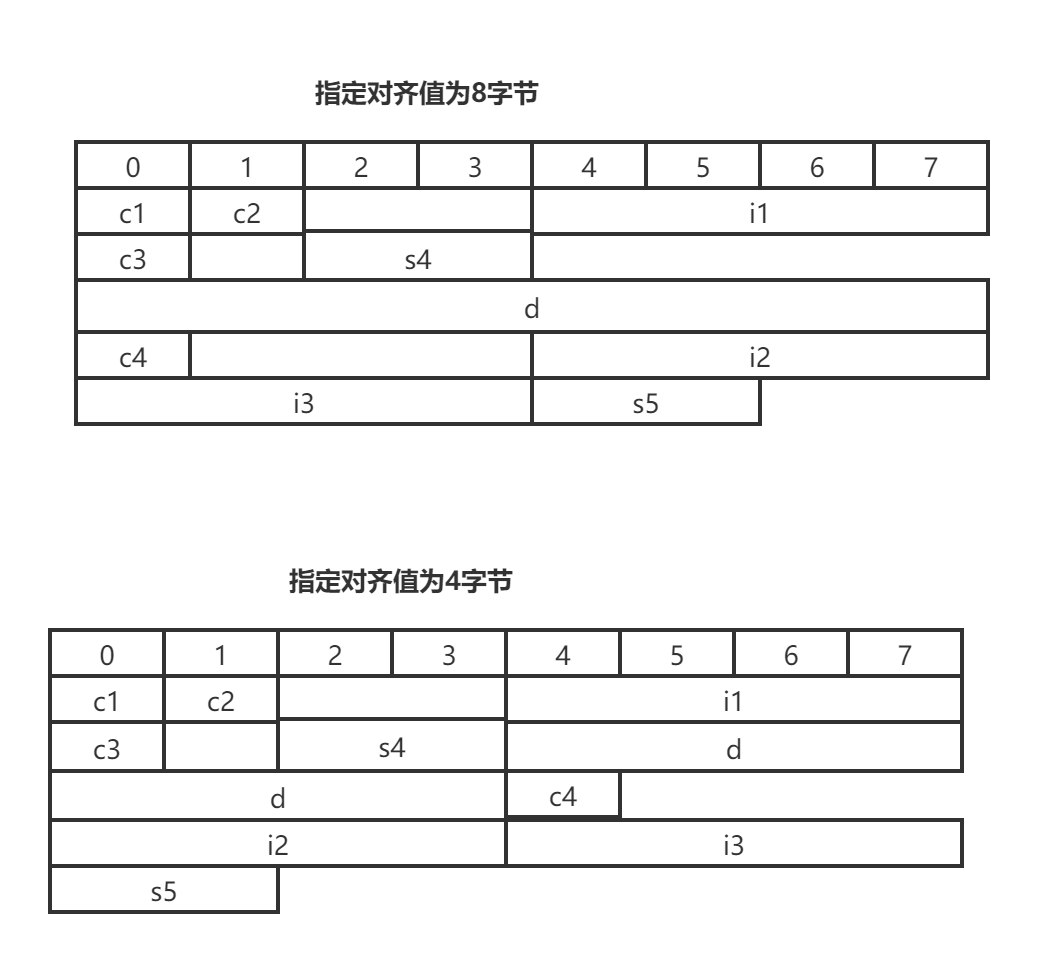### 12.3 公用体(union)

• 成员类型可以是基本数据类型，也可以是构造数据类型。
• 公用体变量初始化时，只能对第一个成员赋值。
• 公用体变量所占的内存长度等于最长的成员长度。
• 公用体变量在一个时刻只能一个成员发挥作用，赋值时，成员之间会互相覆盖，最后一次被赋值的成员起作用。
定义
union 共同体类型名
{
成员类型1 成员名1;
成员类型2 成员名2;
... ...
成员类型n 成员名n;
};


union data
{
int i;
float f;
char c;
}x = {123};
union data
{
float f;
int i;
char c;
};
data x = {12.3};
union
{
char c;
int i;
float f;
}x = {’y‘};


共同体变量名.成员名;
union data
{
int i;
float f;
char c;
}x = {12};
int main()
{
cout << x.i << " " << x.f << " " << x.c << endl;//12 1.68156e-44
x.c = 'c';
cout << x.i <<" "<< x.f << " " << x.c << endl;//99 1.38729e-43 c
return 0;
}


### 12.4 枚举(enum)和typedef声明

typedef-为已存在的数据类型定义一个新的类型名称，不能定义变量
typedef声明格式：typedef 类型名称 类型标识符;

typedef char *CP;
typedef int INTEGER;


# 13 面向对象

### 13.1 类

• 类也是一种数据类型。

class 类名
{
public:
公有数据成员;
公有成员函数;
private:
私有数据成员;
私有成员函数;
protected:
保护数据成员;
保护成员函数;
};


//类外

{
函数体;
}
//内联函数：类外
inline 返回类型 类名:成员函数名(参数列表)
{
函数体;
}


### 13.2 类成员的访问权限以及类的封装

• 和Java、C#不同的是，C++中public、private、protected只能修饰类的成员，不能修饰类，C++中的类没有共有私有之分
• 类内部没有访问权限的限制，都可以互相访问。
• 在C++中用class定义的类中，其成员的默认存取权限是private。

publicYYY
protectedNYY
privateNNY

### 13.3 对象

//1.声明类同时定义对象
class 类名
{
类体;
}对象名列表;
//2.先声明类，再定义对象

//3. 不出现类名，直接定义对象
class
{
类体;
}对象名列表;
//4.在堆上创建对象
Person p(123, "yar");//在栈上创建对象
Person *pp = new Person(234,"yar");//在堆上创建对象


### 13.4 构造函数

• 构造函数名必须与类名相同
• 没有任何返回值和返回类型
• 创建对象自动调用，不需要用户来调用，且只掉用一次
• 类没有定义任何构造函数，编译系统会自动为这个类生成一个默认的无参构造函数
构造函数定义
//1.类中定义 2.类中声明，类外定义
[类名::]构造函数名(参数列表)
{
函数体
}


类名 对象名(参数列表);//参数列表为空时，()可以不写


class Person
{
public:
Person(int = 0,string = "张三");
void show();
private:
int age;
string name;
};
Person::Person(int a, string s)
{
cout<<a<<" "<<s<<endl;
age = a;
name = s;
}
void Person::show()
{
cout << "age="<<age << endl;
cout << "name=" <<name << endl;
}
int main()
{
Person p; //0 张三
Person p2(12);//12 张三
Person p3(123, "yar");//123 yar
return 0;
}


类名::构造函数名(参数列表):参数初始化表
{
函数体;
}

class Person
{
public:
Person(int = 0,string = "张三");
void show();
private:
int age;
string name;
};
Person::Person(int a, string s):age(a),name(s)
{
cout << a << " " << s << endl;
}


class Person
{
public:
Person();
Person(int = 0,string = "张三");
Person(double,string);
void show();
private:
int age;
double height;
string name;
};
...


类名::类名(类名&对象名)
{
函数体;
}
class Person
{
public:
Person(Person &p);//声明拷贝构造函数
Person(int = 0,string = "张三");
void show();
private:
int age;
string name;
};
Person::Person(Person &p)//定义拷贝构造函数
{
cout << "拷贝构造函数" << endl;
age = 0;
name = "ABC";
}
Person::Person(int a, string s):age(a),name(s)
{
cout << a << " " << s << endl;
}
int main()
{
Person p(123, "yar");
Person p2(p);
p2.show();
return 0;
}
//输出
123 yar

age=0
name=ABC


### 13.5 析构函数

• 析构函数名与类名必须相同。
• 析构函数名前面必须加一个波浪号~。
• 没有参数，没有返回值，不能重载。
• 一个类中只能有一个析构函数。
• 没有定义析构函数，编译系统会自动为和这个类生成一个默认的析构函数。
析构函数的定义：
//1.类中定义 2.类中声明，类外定义
[类名::]~析构函数名()
{
函数体;
}


### 13.6 对象指针

类名 *对象指针名;

//访问对象成员

Person p(123, "yar");
Person* pp = &p;
Person* pp2 = new Person(234,"yar")
pp->show();


数据成员类型 *指针变量名 = &对象名.数据成员名;

(对象名.*指针变量名)(参数列表);
Person p(123, "yar");
void(Person::*pfun)();
pfun = &Person::show;
(p.*pfun)();


this指针

class Person
{
public:
Person(int = 0,string = "张三");
void show();
private:
int age;
string name;
};
Person::Person(int a, string s):age(a),name(s)
{
cout << a << " " << s << endl;
}
void Person::show()
{
cout << "age="<<this->age << endl;
cout << "name=" <<this->name << endl;
}


### 13.7 静态成员

• static 成员变量属于类，不属于某个具体的对象
• 静态成员函数只能访问类中静态数据成员
静态数据成员
//类内声明，类外定义
class xxx
{
static 数据类型 静态数据成员名;
}

//访问



//类内声明，类外定义
class xxx
{
static 返回值类型 静态成员函数名(参数列表);
}

{
函数体;
}
//访问



### 13.8 友元

• 友元函数不是类的成员函数，所以没有this指针，必须通过参数传递对象。
• 友元函数中不能直接引用对象成员的名字，只能通过形参传递进来的对象或对象指针来引用该对象的成员。
//1.将非成员函数声明为友元函数
class Person
{
public:
Person(int = 0,string = "张三");
friend void show(Person *pper);//将show声明为友元函数
private:
int age;
string name;
};
Person::Person(int a, string s):age(a),name(s)
{
cout << a << " " << s << endl;
}
void show(Person *pper)
{
cout << "age="<< pper->age << endl;
cout << "name=" << pper->name << endl;
}
int main()
{;
Person *pp = new Person(234,"yar");
show(pp);
system("pause");
return 0;
}
//2.将其他类的成员函数声明为友元函数
//person中的成员函数可以访问MobilePhone中的私有成员变量
class MobilePhone;//提前声明
//声明Person类
class Person
{
public:
Person(int = 0,string = "张三");
void show(MobilePhone *mp);
private:
int age;
string name;
};
//声明MobilePhone类
class MobilePhone
{
public:
MobilePhone();
friend void Person::show(MobilePhone *mp);
private:
int year;
int memory;
string name;
};
MobilePhone::MobilePhone()
{
year = 1;
memory = 4;
name = "iphone 6s";
}
Person::Person(int a, string s):age(a),name(s)
{
cout << a << " " << s << endl;
}
void Person::show(MobilePhone *mp)
{
cout << mp->year << "年  " << mp->memory << "G " << mp->name << endl;
}
int main()
{
Person *pp = new Person(234,"yar");
MobilePhone *mp = new MobilePhone;
pp->show(mp);
system("pause");
return 0;
}


• 类之间的友元关系不能传递
• 类之间的友元关系是单向的
• 友元关系不能被继承
class HardDisk
{
public:
HardDisk();
friend class Computer;
private:
int capacity;
int speed;
string brand;
};
HardDisk::HardDisk():capacity(128),speed(0),brand("三星"){
}
class Computer
{
public:
Computer(HardDisk hd);
void start();
private:
string name;
int ram;
string cpu;
int osType;
HardDisk hardDisk;

};
{
cout << "正在创建computer..." << endl;
this->hardDisk = hd;
this->hardDisk.speed = 5400;
cout << "硬盘转动...speed = " << this->hardDisk.speed << "转/分钟" << endl;

}
void Computer::start()
{

cout << hardDisk.brand << " " << hardDisk.capacity << "G" << hardDisk.speed << "转/分钟" << endl;
cout << "笔记本开始运行..." << endl;
}
int main()
{
HardDisk hd;
Computer cp(hd);
cp.start();
system("pause");
return 0;
}


### 13.9 类(class)与结构体(struct)的区别

• 引入C语言的结构体，是为了保证和c程序的兼容性。
• c语言中的结构体不允许定义函数成员，且没有访问控制权限的属性。
• c++为结构体引入了成员函数，访问控制权限，继承，多态等面向对象特性。
• c语言中，空结构体的大小为0，而C++中空结构体大小为1。
• class中成员默认是private,struct中的成员默认是public。
• class继承默认是private继承，而struct继承默认是public继承。
• class可以使用模版，而struct不能。
举个例子：
//结构体默认权限为public
struct person
{
void show();
string name;
int age;
};
int main()
{
person p;
p.name = "heiren";
p.age = 666;
p.show();
cout <<"name="<< p.name <<" age="<< p.age << endl;
system("pause");
return 0;
}


# 14 继承和派生

### 14.1 继承和派生概述

• 一个基类可以派生出多个派生类，一个派生类可以继承多个基类
派生类的声明：
//继承方式为可选项，默认为private，还有public,protected
class 派生类名：[继承方式]基类名
{
派生类新增加的成员声明;
};


• public-基类的public成员和protected成员的访问属性保持不变，私有成员不可见。
• private-基类的public成员和protected成员成为private成员,只能被派生类的成员函数直接访问，私有成员不可见。
• protected-基类的public成员和protected成员成为protected成员,只能被派生类的成员函数直接访问，私有成员不可见。

publicpublicprotected不可见
protectedprotectedprotected不可见
privateprivateprivate不可见
• 利用using关键字可以改变基类成员再派生类中的访问权限；using只能修改基类中public和protected成员的访问权限。
class Base
{
public:
void show();
protected:
int aa;
double dd;
};
void Base::show(){
}
class Person:public Base
{
public:
using Base::aa;//将基类的protected成员变成public
using Base::dd;//将基类的protected成员变成public
private:
using Base::show;//将基类的public成员变成private
string name;
};
int main()
{
Person *p = new Person();
p->aa = 12;
p->dd = 12.3;
p->show();//出错
delete p;
return 0;
}


• 先执行基类的构造函数，随后执行派生类的构造函数
• 先执行派生类的析构函数，再执行基类的析构函数。
• 派生类的构造函数：派生类名(总参数列表)：基类名(基类参数列表),子对象名1(参数列表){构造函数体;}
class Base
{
public:
Base(int, double);
~Base();
private:
int aa;
double dd;
};
Base::Base(int a, double d) :aa(a), dd(d)
{
cout << "Base Class 构造函数!!!" << endl;
}
Base::~Base()
{
cout << "Base Class 析构函数!!!" << endl;
}
class Person:public Base
{
public:
Person(int,double,string);
~Person();
private:
string name;
};
Person::Person(int a,double d,string str):Base(a,d),name(str)
{
cout << "Person Class 构造函数!!!" << endl;
}
Person::~Person()
{
cout << "Person Class 析构函数!!!" << endl;
}
int main()
{
cout << "创建Person对象..." << endl;
Person *p = new Person(1,2,"yar");
cout << "删除Person对象...." << endl;
delete p;
system("pause");
return 0;
}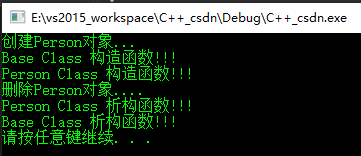### 14.2 多继承

class 派生类名:继承方式1 基类1,继承方式2 基类2
{
派生类主体;
};


派生类名(总参数列表)：基类名1(基类参数列表1),基类名2(基类参数列表2),

{
构造函数体;
}


• 1.类名::同名成员名;
• 2.派生类定义同名成员，访问的就是派生类同名成员。

### 14.3 虚基类

c++引入虚基类使得派生类再继承间接共同基类时只保留一份同名成员。

• 虚继承的目的是让某个类做出声明，承诺愿意共享它的基类。其中，这个被共享的基类就称为虚基类（Virtual Base Class）。
• 派生类的 同名成员 比虚基类的 优先级更高
虚基类的声明：class 派生类名:virtual 继承方式 基类名
class  A//虚基类
{
protected:
int a;
};
class B: virtual public A
{
protected:
int b;
};
class C:virtual public A
{
protected:
int c;
};
class D:public B,public C
{
protected:
int d;
void show()
{
b = 123;
c = 23;
a = 1;
}
};

• 如果 B 或 C 其中的一个类定义了a，也不会有二义性，派生类的a 比虚基类的a 优先级更高。
• 如果 B 和 C 中都定义了 a，那么D直接访问a 将产生二义性问题。
应用：c++中的iostream ， istream ， ostream，base_io

# 15 多态和虚函数

### 15.1 向上转型

int a = 66.9;
printf("%d\n", a);//66
float b = 66;
printf("%f\n", b);//66.000000

• 只能将将派生类赋值给基类（C++中称为向上转型）： 派生类对象赋值给基类对象、将派生类指针赋值给基类指针、将派生类引用赋值给基类引用
• 派生类对象赋值给基类对象，舍弃派生类新增的成员；派生类指针赋值给基类指针，没有拷贝对象的成员，也没有修改对象本身的数据，仅仅是改变了指针的指向；派生类引用赋值给基类引用，和指针的一样。、

### 15.2 多态

• 静态多态性-在程序编译时系统就决定调用哪个函数，比如函数重载和静态多态性
• 动态多态性-在程序运行过程中动态确定调用那个函数，通过虚函数实现的。

### 15.3 虚函数

• 将基类中的函数声明为虚函数，派生类中的同名函数自动为虚函数。
• 声明形式：virtual 函数类型 函数名 (参数列表);
• 构造函数不能声明为虚函数，析构函数可以声明为虚函数。
class  A
{
public:
virtual void show()
{
cout << "A show" << endl;
}
};
class B:  public A
{
public:
void show()
{
cout << "B show" << endl;
}
};
int main()
{

B b;
b.show();//B show
A *pA = &b;
pA->show();//B show 如果show方法前没用virtual声明为虚函数，这里会输出A show

system("pause");
return 0;
}


### 15.4 纯虚函数

class  A
{
public:
virtual void show() = 0;
};
class B:  public A
{
public:
void show()
{
cout << "B show" << endl;
}
};


# 16 运算符重载

• 运算符重载是通过函数实现的，它本质上是函数重载。

• 不允许重载的运算符

### 16.1 定义

• 不可以自己定义新的运算符，只能对已有的C++运算符重载。
• 不能改变运算符运算对象的个数。
• 不能改变运算符的优先级和结合性
• 应与标准类型运算功能相似，避免影响可读性。
一般格式：
函数类型 operator运算符(参数列表)
{
函数体
}
//举个栗子：定义一个向量类，通过运算符重载，可以用+进行运算。
class Vector3
{
public:
Vector3();
Vector3(double x,double y,double z);
public:
Vector3 operator+(const Vector3 &A)const;
void display()const;
private:
double m_x;
double m_y;
double m_z;
};
Vector3::Vector3() :m_x(0.0), m_y(0.0), m_z(0.0) {}
Vector3::Vector3(double x, double y,double z) : m_x(x), m_y(y), m_z(z) {}
//运算符重载
Vector3 Vector3::operator+(const Vector3 &A) const
{
Vector3 B;
B.m_x = this->m_x + A.m_x;
B.m_y = this->m_y + A.m_y;
B.m_z = this->m_z + A.m_z;
return B;
}
void  Vector3::display()const
{
cout<<"(" << m_x << "," << m_y << "," << m_z << ")" << endl;
}


### 16.2 形式

• 双目运算符作为友元函数时需要制定两个参数。
• 运算符重载函数作为类成员函数可以显式调用。
class Vector3
{
public:
Vector3();
Vector3(double x,double y,double z);
public:
Vector3 operator+(const Vector3 &A)const;
Vector3 operator++();
friend Vector3 operator-(const Vector3 &v1, const Vector3 &v2);
friend Vector3 operator--(Vector3 &v);
void display()const;
private:
double m_x;
double m_y;
double m_z;
};
Vector3::Vector3() :m_x(0.0), m_y(0.0), m_z(0.0) {}
Vector3::Vector3(double x, double y,double z) : m_x(x), m_y(y), m_z(z) {}
//运算符重载
Vector3 Vector3::operator+(const Vector3 &A) const
{
Vector3 B;
B.m_x = this->m_x + A.m_x;
B.m_y = this->m_y + A.m_y;
B.m_z = this->m_z + A.m_z;
return B;
}
Vector3 Vector3::operator++()
{
this->m_x ++;
this->m_y ++;
this->m_z ++;
return *this;
}
void  Vector3::display()const
{
cout<<"(" << m_x << "," << m_y << "," << m_z << ")" << endl;
}
Vector3 operator-(const Vector3 &v1,const Vector3 &v2)
{
Vector3 B(v1.m_x - v2.m_x, v1.m_y - v2.m_y, v1.m_z - v2.m_z);
return B;
}
Vector3 operator--( Vector3 &v)
{
v.m_x--;
v.m_y--;
v.m_z --;
return v;
}
int main()
{
Vector3 v1(1, 2, 3);
Vector3 v2(2, 3, 2);

++v1;//v1.operator++(); 作为类成员函数可以显式调用
v1.display();
--v2;
v2.display();
Vector3 v3 = v1 + v2;// v1.operator+(v2);作为类成员函数可以显式调用
v3.display();
Vector3 v4 = v1 - v2;
v4.display();
return 0;
}


### 16.3 常用运算符的重载

1.自增自减：

//前置运算符 ++a --a
operator++()
operator--()
operator++(Vector3 &v)
operator--(Vector3 &v)
//后置运算符 a-- a++
operator++(int)
operator--(int)
operator++(Vector3 &v,int)
operator--(Vector3 &v,int)


2.赋值运算符：

String& String::operator=(String &s)
{
if(this!=&s)
{
delete[] str;
int length = strlen(s.str);
str = new char[length+1];
strcpy(str,s.str);
}
return (*this)
}


3.输入\输出运算符重载

 friend ostream &operator<<( ostream &output,
const Vector3 &v )
{
output << "F : " <<v.m_x<< " I : " << v.m_y<<v.m_z;
return output;
}

friend istream &operator>>( istream  &input, Vector3 &v )
{
input >> v.m_x>> v.m_y>>v.m_z;
return input;
}


### 16.4 实现类型转换

• 不指定函数类型和参数，返回值的类型由类型名来确定。
• 类型转换函数只能作为成员函数，不能作为友元函数。
类型转换函数的一般形式：
operator 类型名()
{
转换语句;
}
class Vector3
{
public:
Vector3();
Vector3(double x,double y,double z);
public:
Vector3 operator+(const Vector3 &A)const;
Vector3 operator++();
friend Vector3 operator-(const Vector3 &v1, const Vector3 &v2);
friend Vector3 operator--(Vector3 &v,int);
operator double()
{
return m_x + m_y + m_z;
}
void display()const;
private:
double m_x;
double m_y;
double m_z;
};
int main()
{
Vector3 v1(1, 2, 3);
double d = v1;
cout << d << endl;//6
return 0;
}


# 17 IO流

### 17.1 流类和对象

• 输入流-从输入设备流向内存的流。
• 输出流-从内存流出设备的流。
• 内存缓冲区-用来存放流中的数据。

输出缓冲区=(缓冲满或endl)》‘<<’送到 显示器显示

iostream：ios ,istream,ostream,iostream
fstream：ifstream,ofstream,fstream
strstream：istrstream,ostrstream,strstream

• istream 是用于输入的流类，cin 就是该类的对象。
• ostream 是用于输出的流类，cout 就是该类的对象。
• ifstream 是用于从文件读取数据的类。
• ofstream 是用于向文件写入数据的类。
• iostream 是既能用于输入，又能用于输出的类。
• fstream 是既能从文件读取数据，又能向文件写入数据的类。
• istrstream 输入字符串类
• ostrstream 输出字符串类
• strstream 输入输出字符串流类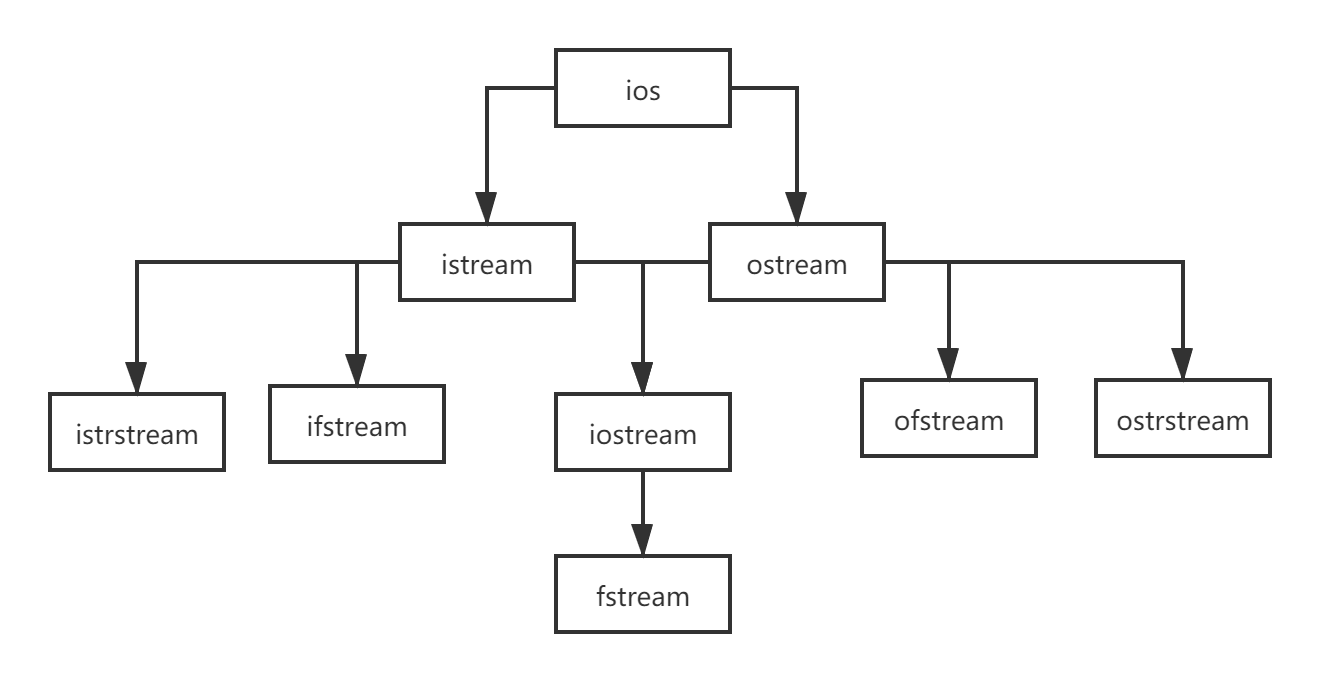### 17.2 标准输入输出流

C++的输入/输出流库(iostream)中定义了4个标准流对象：cin(标准输入流-键盘)，cout(标准输出流-屏幕)，cerr(标准错误流-屏幕)，clog(标准错误流-屏幕)

• cerr 不使用缓冲区，直接向显示器输出信息；而输出到 clog 中的信息会先被存放到缓冲区，缓冲区满或者刷新时才输出到屏幕。
• cout 是 ostream 类的对象，ostream 类的无参构造函数和复制构造函数都是私有的，所以无法定义 ostream 类的对象。
• 使用>>提取数据时，系统会跳过空格，制表符，换行符等空白字符。所以一组变量输入值时，可用这些隔开。
• 输入字符串，也是跳过空白字符，会在串尾加上字符串结束标志\0。
int  x;
double y;
cin>>x>>y;
//输入 22 66.0  两个数之间可以用空格、制表符和回车分隔数据
char str;
cin>>str;//hei ren  字符串中只有hei\0


• get函数：cin.get()，cin.get(ch)（成功返回非0值，否则返回0），cin.get(字符数组(或字符指针)，字符个数n,终止字符)
char c = cin.get();//获取一个字符
while ((c = cin.get()) != EOF)//循环读取，直到换行
{
cout << c;
}

char ch;
cin.get(ch);
while (cin.get(ch))//读取成功循环
{
cout << ch;
}

char arr;
cin.get(arr, 5, '\n');//输入 heiren  结果 heir\0

• getline函数：cin.getline(字符数组(或字符指针),字符个数n,终止标志字符)
读取字符知道终止字符或者读取n-1个字符，赋值给指定字符数组（或字符指针）
char arr0,arr1,arr2;
cin>>arr0;//遇到空格、制表符或回车结束  "Heiren"
cin.getline(arr1,30);//字符数最多为29个，遇到回车结束 " Hello World"
cin.getline(arr2,40,'*');//最多为39个，遇到*结束 "yar"
//输入 Heiren Hello World
//yar*123

• cin.peek() 不会跳过输入流中的空格、回车符。在输入流已经结束的情况下，cin.peek() 返回 EOF。
• ignore(int n =1, int delim = EOF)
int n;
cin.ignore(5, 'Y');//跳过前5个字符或Y之前的字符，‘Y’优先
cin >> n;
//输入1234567  ->  67    1234567Y345->345

//输入2020.2.23
int year,month,day;
cin >> year ;
cin.ignore() >> month ; //用ignore跳过 '.'
cin.ignore() >> day;
cin.ignore();   //跳过行末 '\n'

cout<< setfill('0') << setw(2) << month ;//设置填充字符'\0'，输出宽度2
cout << "-" << setw(2) << day << "-" << setw(4) << year << endl;

• putback(char c)，可以将一个字符插入输入流的最前面。

• 插入endl-输出所有数据，插入换行符，清空缓冲区
• \n-输出换行，不清空缓冲区
• cout.put(参数) 输出单个字符（可以时字符也可以是ASII码）

iomanip 中定义的流操作算子：

• *不是算子的一部分，星号表示在没有使用任何算子的情况下，就等效于使用了该算子，例如，在默认情况下，整数是用十进制形式输出的，等效于使用了 dec 算子

*dec以十进制形式输出整数 常用
hex以十六进制形式输出整数
oct以八进制形式输出整数
fixed以普通小数形式输出浮点数
scientific以科学计数法形式输出浮点数
left左对齐，即在宽度不足时将填充字符添加到右边
*right右对齐，即在宽度不足时将填充字符添加到左边
setbase(b)设置输出整数时的进制，b=8、10 或 16
setw(w)指定输出宽度为 w 个字符，或输人字符串时读入 w 个字符
setprecision(n)设置输出浮点数的精度为 n。在使用非 fixed 且非 scientific 方式输出的情况下，n 即为有效数字最多的位数，如果有效数字位数超过 n，则小数部分四舍五人，或自动变为科学计 数法输出并保留一共 n 位有效数字。在使用 fixed 方式和 scientific 方式输出的情况下，n 是小数点后面应保留的位数。
setiosflags(flag)将某个输出格式标志置为 1
resetiosflags(flag)将某个输出格式标志置为 0
boolapha把 true 和 false 输出为字符串 不常用
*noboolalpha把 true 和 false 输出为 0、1
showbase输出表示数值的进制的前缀
*noshowbase不输出表示数值的进制.的前缀
showpoint总是输出小数点
*noshowpoint只有当小数部分存在时才显示小数点
showpos在非负数值中显示 +
*noshowpos在非负数值中不显示 +
*skipws输入时跳过空白字符
noskipws输入时不跳过空白字符
uppercase十六进制数中使用 A~E。若输出前缀，则前缀输出 0X，科学计数法中输出 E
*nouppercase十六进制数中使用 a~e。若输出前缀，则前缀输出 0x，科学计数法中输出 e。
internal数值的符号（正负号）在指定宽度内左对齐，数值右对 齐，中间由填充字符填充。
• 流操作算子使用方法：cout << hex << 12 << "," << 24;//c,18

setiosflags() 算子
setiosflags() 算子实际上是一个库函数，它以一些标志作为参数，这些标志可以是在 iostream 头文件中定义的以下几种取值，它们的含义和同名算子一样。

ios::left输出数据在本域宽范围内向左对齐
ios::right输出数据在本域宽范围内向右对齐
ios::internal数值的符号位在域宽内左对齐，数值右对齐，中间由填充字符填充
ios::dec设置整数的基数为 10
ios::oct设置整数的基数为 8
ios::hex设置整数的基数为 16
ios::showbase强制输出整数的基数（八进制数以 0 开头，十六进制数以 0x 打头）
ios::showpoint强制输出浮点数的小点和尾数 0
ios::uppercase在以科学记数法格式 E 和以十六进制输出字母时以大写表示
ios::showpos对正数显示“+”号
ios::scientific浮点数以科学记数法格式输出
ios::fixed浮点数以定点格式（小数形式）输出
ios::unitbuf每次输出之后刷新所有的流
ios::stdio每次输出之后清除 stdout, stderr
• 多个标志可以用|运算符连接，表示同时设置。例如：
cout << setiosflags(ios::scientific|ios::showpos) << 12.34;//+1.234000e+001

• 如果两个相互矛盾的标志同时被设置,结果可能就是两个标志都不起作用,应该用 resetiosflags 清除原先的标志
cout << setiosflags(ios::fixed) << 12.34 << endl;
cout << resetiosflags(ios::fixed) << setiosflags(ios::scientific | ios::showpos) << 12.34 << endl;


ostream 类中的成员函数：

precision(n)setprecision(n)设置输出浮点数的精度为 n。
width(w)setw(w)指定输出宽度为 w 个字符。
setf(flag)setiosflags(flag)将某个输出格式标志置为 1。
unsetf(flag)resetiosflags(flag)将某个输出格式标志置为 0。
• setf 和 unsetf 函数用到的flag，与 setiosflags 和 resetiosflags 用到的完全相同。
cout.setf(ios::scientific);
cout.precision(8);
cout << 12.23 << endl;//1.22300000e+001


# 18 文件操作

• ASCII文件输出还是二进制文件，数据形式一样，对于数值数据，输出不同

### 18.1 文件类和对象

C++ 标准类库中有三个类可以用于文件操作，它们统称为文件流类。这三个类是：

• ifstream：输入流类，用于从文件中读取数据。
• ofstream：输出流类，用于向文件中写人数据。
• fstream：输入/输出流类，既可用于从文件中读取数据，又可用于 向文件中写人数据。
文件流对象定义：
#include <fstream>
ifstream in;
ofstream out;
fstream inout;


### 18.2 打开文件

• 打开文件的目的：建立对象与文件的关联，指明文件使用方式
• 打开文件的两种方式：open函数和构造函数

open函数：void open(const char* szFileName, int mode);

ios::inifstream fstream打开文件用于读取数据。如果文件不存在，则打开出错。
ios::outofstream fstream打开文件用于写入数据。如果文件不存在，则新建该文件；如 果文件原来就存在，则打开时清除原来的内容。
ios::appofstream fstream打开文件，用于在其尾部添加数据。如果文件不存在，则新建该文件。
ios::ateifstream打开一个已有的文件，并将文件读指针指向文件末尾（读写指 的概念后面解释）。如果文件不存在，则打开出错。
ios:: truncofstream单独使用时与 ios:: out 相同。
ios::binaryifstream ofstream fstream以二进制方式打开文件。若不指定此模式，则以文本模式打开。
ios::in | ios::outfstream打开已存在的文件，既可读取其内容，也可向其写入数据。文件刚打开时，原有内容保持不变。如果文件不存在，则打开出错。
ios::in | ios::outofstream打开已存在的文件，可以向其写入数据。文件刚打开时，原有内容保持不变。如果文件不存在，则打开出错。
ios::in | ios::out | ios::truncfstream打开文件，既可读取其内容，也可向其写入数据。如果文件本来就存在，则打开时清除原来的内容；如果文件不存在，则新建该文件。

ios::binary 可以和其他模式标记组合使用，例如：

• ios::in | ios::binary表示用二进制模式，以读取的方式打开文件。
• ios::out | ios::binary表示用二进制模式，以写入的方式打开文件。

eg:ifstream::ifstream (const char* szFileName, int mode = ios::in, int);

#include <iostream>
#include <fstream>
using namespace std;
int main()
{
ifstream inFile("c:\\tmp\\test.txt", ios::in);
if (inFile)
inFile.close();
else
cout << "test.txt doesn't exist" << endl;
ofstream oFile("test1.txt", ios::out);
if (!oFile)
cout << "error 1";
else
oFile.close();
fstream oFile2("tmp\\test2.txt", ios::out | ios::in);
if (!oFile2)
cout << "error 2";
else
oFile.close();
return 0;
}


### 18.3 文本文件的读写

eg：编写一个程序，将文件 i.txt 中的整数倒序输出到 o.txt。（12 34 56 78 90 -> 90 78 56 34 12）

#include <iostream>
#include <fstream>
using namespace std;
int arr;
int main()
{

int num = 0;
ifstream inFile("i.txt", ios::in);//文本模式打开
if (!inFile)
return 0;//打开失败
ofstream outFile("o.txt",ios::out);
if (!outFile)
{
outFile.close();
return 0;
}
int x;
while (inFile >> x)
arr[num++] = x;
for (int i = num - 1; i >= 0; i--)
outFile << arr[i] << " ";
inFile.close();
outFile.close();
return 0;
}



### 18.4 二进制文件的读写

• 用文本方式存储信息不但浪费空间，而且不便于检索。
• 二进制文件中，信息都占用 sizeof(对象名) 个字节；文本文件中类的成员数据所占用的字节数不同，占用空间一般比二进制的大。

ostream::write 成员函数：ostream & write(char* buffer, int count);

class Person
{
public:
char m_name;
int m_age;
};
int main()
{

Person p;
ofstream outFile("o.bin", ios::out | ios::binary);
while (cin >> p.m_name >> p.m_age)
outFile.write((char*)&p, sizeof(p));//强制类型转换
outFile.close();
//heiren 烫烫烫烫烫烫啼
return 0;
}


istream::read 成员函数：istream & read(char* buffer, int count);

Person p;
ifstream inFile("o.bin", ios::in | ios::binary); //二进制读方式打开
if (!inFile)
return 0;//打开文件失败
cout << p.m_name << " " << p.m_age << endl;
inFile.close();


#include <iostream>
#include <fstream>
using namespace std;
int main()
{

ifstream inFile("a.txt", ios::binary | ios::in);
if (!inFile)
return 0;
ofstream outFile("b.txt", ios::binary | ios::out);
if (!outFile)
{
inFile.close();
return 0;
}
char c;
while (inFile.get(c))  //每读一个字符
outFile.put(c);  //写一个字符
outFile.close();
inFile.close();
return 0;
}


### 18.5 移动和获取文件读写指针

• ifstream 类和 fstream 类有 seekg 成员函数，可以设置文件读指针的位置；
• ofstream 类和 fstream 类有 seekp 成员函数，可以设置文件写指针的位置。
• ifstream 类和 fstream 类还有 tellg 成员函数，能够返回文件读指针的位置；
• ofstream 类和 fstream 类还有 tellp 成员函数，能够返回文件写指针的位置。

ostream & seekp (int offset, int mode);
istream & seekg (int offset, int mode);
//mode有三种：ios::beg-开头往后offset(>=0)字节 ios::cur-当前往前(<=0)/后(>=0)offset字节 ios::end-末尾往前(<=0)offect字节
int tellg();
int tellp();
//seekg 函数将文件读指针定位到文件尾部，再用 tellg 函数获取文件读指针的位置，此位置即为文件长度


#include <iostream>
#include <fstream>
//#include <vector>
//#include<cstring>
using namespace std;

class Person
{
public:
char m_name;
int m_age;
};
int main()
{

Person p;
ifstream ioFile("p.bin", ios::in | ios::out);//用既读又写的方式打开
if (!ioFile)
return 0;
ioFile.seekg(0, ios::end); //定位读指针到文件尾部，以便用以后tellg 获取文件长度
int L = 0, R; // L是折半查找范围内第一个记录的序号
// R是折半查找范围内最后一个记录的序号
R = ioFile.tellg() / sizeof(Person) - 1;
do {
int mid = (L + R) / 2;
ioFile.seekg(mid *sizeof(Person), ios::beg);
int tmp = strcmp(p.m_name, "Heiren");
if (tmp == 0)
{
cout << p.m_name << " " << p.m_age;
break;
}
else if (tmp > 0)
R = mid - 1;
else
L = mid + 1;
} while (L <= R);
ioFile.close();

system("pause");
return 0;
}


### 18.6 文本文件和二进制文件打开方式的区别

• UNIX/Linux 平台中，用文本方式或二进制方式打开文件没有任何区别。
• 在 UNIX/Linux 平台中，文本文件以\n（ASCII 码为 0x0a）作为换行符号；而在 Windows 平台中，文本文件以连在一起的\r\n（\r的 ASCII 码是 0x0d）作为换行符号。
• 在 Windows 平台中，如果以文本方式打开文件，当读取文件时，系统会将文件中所有的\r\n转换成一个字符\n，如果文件中有连续的两个字节是 0x0d0a，则系统会丢弃前面的 0x0d 这个字节，只读入 0x0a。当写入文件时，系统会将\n转换成\r\n写入。

# 19 泛型和模板

• 泛型程序设计在实现时不指定具体要操作的数据的类型的程序设计方法的一种算法，指的是算法只要实现一遍，就能适用于多种数据类型，优势在于代码复用，减少重复代码的编写。
• 模板是泛型的基础，是创建泛型类或函数的蓝图或公式。

### 19.1 函数模板

template<class T>或template<typename T>

{
函数体;
}
template<class T1,class T2,...>//class可以换成typename

{
函数体;
}
//举个栗子
template<class T> T max(T a, T b)
{
return a > b ? a : b;
}
int main()
{
cout <<"max value is "<< max(12,34) << endl;//34
cout << "max value is " << max(12.4, 13.6) << endl;//13.6
cout << "max value is " << max(12.4, 13) << endl;//error 没有与参数列表匹配的 函数模板 "max" 实例参数类型为:(double, int)
return 0;
}


### 19.2 类模板

• 声明了类模板，就可以将类型参数用于类的成员函数和成员变量了。换句话说，原来使用 int、float、char 等内置类型的地方，都可以用类型参数来代替。
类模板的一般形式：
template<class T>//class可以换成typename 模板头
class 类名
{
函数定义;
};
//多个类型参数和函数模板类似，逗号隔开


template<class T>//class可以换成typename

{
函数体;
}


template<typename T1, typename T2>  // 模板头  没有分号
class Point {
public:
Point(T1 x, T2 y) : x(x), y(y) { }
public:
T1 getX() const;  //成员函数后加const，声明该函数内部不会改变成员变量的值
void setX(T1 x);
T2 getY() const;
void setY(T2 y);
private:
T1 x;
T2 y;
};
template<typename T1, typename T2>  //模板头
T1 Point<T1, T2>::getX() const  {
return x;
}
template<typename T1, typename T2>
void Point<T1, T2>::setX(T1 x) {
x = x;
}
template<typename T1, typename T2>
T2 Point<T1, T2>::getY() const {
return y;
}
template<typename T1, typename T2>
void Point<T1, T2>::setY(T2 y) {
y = y;
}
int main()
{
Point<int, double> p1(66, 20.5);
Point<int, char*> p2(10, "东经33度");
Point<char*, char*> *p3 = new Point<char*, char*>("西经12度", "北纬66度");
cout << "x=" << p1.getX() << ", y=" << p1.getY() << endl;
cout << "x=" << p2.getX() << ", y=" << p2.getY() << endl;
cout << "x=" << p3->getX() << ", y=" << p3->getY() << endl;
return 0;
}


### 19.3 typename 和 class 的区别

在模板引入 c++ 后，采用class来定义模板参数类型，后来为了避免 class 在声明类和模板的使用可能给人带来混淆，所以引入了 typename 这个关键字。

• 模板定义语法中关键字 class 与 typename 的作用完全一样。
• 不同的是typename 还有另外一个作用为：使用嵌套依赖类型(nested depended name)
class MyClass
{
public:
typedef int LengthType;
LengthType getLength() const
{
return this->length;
}
void setLength(LengthType length)
{
this->length = length;
}

private:
LengthType length;
};
template<class T>
void MyMethod(T myclass)
{
//告诉 c++ 编译器，typename 后面的字符串为一个类型名称，而不是成员函数或者成员变量
typedef typename T::LengthType LengthType; //
LengthType length = myclass.getLength();
cout << "length = " <<length<< endl;

}
int main()
{
MyClass my;
my.setLength(666);
MyMethod(my);//length = 666
return 0;
}


### 19.4 强弱类型语言和c++模板的那点猫腻

• 强类型语言-在定义变量时需要显式地指明数据类型，为变量指明某种数据类型后就不能赋予其他类型的数据了，除非经过强制类型转换或隐式类型转换。典型的强类型语言有 C/C++、Java、C# 等。
int a = 123;  //不转换
a = 12.89;  //隐式转换 12（舍去小数部分）
a = (int)"heiren,HelloWorld";  //强制转换（得到字符串的地址） 不同类型之间转换需要强制

//Java 对类型转换的要求比 C/C++ 更为严格，隐式转换只允许由低向高转，由高向低转必须强制转换。
int a = 100;  //不转换
a = (int)12.34;  //强制转换（直接舍去小数部分，得到12）

• 弱类型语言-在定义变量时不需要显式地指明数据类型，编译器（解释器）会根据赋给变量的数据自动推导出类型，并且可以赋给变量不同类型的数据。典型的弱类型语言有 JavaScript、Python、PHP、Ruby、Shell、Perl 等。
var a = 100;  //赋给整数
a = 12.34;  //赋给小数
a = "heiren,HelloWorld";  //赋给字符串
a = new Array("JavaScript","React","JSON");  //赋给数组


强类型语言在编译期间就能检测某个变量的操作是否正确，因为变量的类型始终哦都市确定的，加快了程序的运行；对于弱类型的语言，变量的类型可以随时改变，编译器在编译期间能确定变量的类型，只有等到程序运行后、赋值后才能确定变量当前是什么类型，所以传统的编译对弱类型语言意义不大。

• 解释型语言-弱类型往往是解释型语言，边执行边编译
• 编译型语言-先编译后执行。

强类型语言较为严谨，在编译时就能发现很多错误，适合开发大型的、系统级的、工业级的项目；而弱类型语言较为灵活，编码效率高，部署容易，学习成本低，在 Web 开发中大显身手。另外，强类型语言的 IDE 一般都比较强大，代码感知能力好，提示信息丰富；而弱类型语言一般都是在编辑器中直接书写代码。

C++模板退出的动力来源是对数据结构的封装：数据结构关注的是数据的存储以及对其的增删改查操作，C++开发者们想封装这些结构，但是这些结构中数据成分的类型无法提前预测，于是模板诞生了。

STL(Standard Template Library，标准模板库)就是c++对数据结构封装后的称呼。

# 20 命名空间和异常处理

### 20.1 命名空间

命名空间实际上是由用户自己命名的一块内存区域，用户可以根据需要指定一个有名字的空间区域，每个命名空间都有一个作用域，将一些全局实体放在该命名空间中，就与其他全局实体分割开来。

namespace [命名空间名]//名省略时，表示无名的命名空间
{
命名空间成员;
}


• using声明后，在using语句所在的作用域中使用该命名空间成员时，不必再用命名空间名加以限定。
• 标准C++库的所有标识符（包括函数、类、对象和类模板）都是在一个名为std的命名空间中定义的。
• 无名的命名空间,只在本文件的作用域内有效。

### 20.2 异常处理

异常就是程序在执行过程中，由于使用环境变化和用户操作等原因产生的错误，从而影响程序的运行。

• 程序中常见的错误：语法错误，运行错误
• 异常处理机制的组成：检查异常（try）、抛出异常（throw）、捕获并处理异常（catch）

• 被检查的语句必须放在try后面的{}中，否则不起作用，{}不能省略。
• 进行异常处理的语句必须放在catch后面的{}中，catch后()中的异常信息类型不能省略，变量名可以省略。
• catch语句块不能单独使用，必须和try语句块作为整体出现。
• 在try-catch结构中，只能有一个try，但可以有多个catch.
• catch(…)通常放在最后，可以捕获任何类型的异常信息。
try
{
被检查的语句（可以有多个throw语句）;
}
catch(异常信息类型 [变量名])
{

}


throw语句：thow 变量或表达式;

• throw放在try中，也可以单独使用，向上一层函数寻找try-catch，没有找到系统就会调用系统函数terminate，使程序终止运行。
try
{
throw 123;
}
catch (int a)
{
cout << a << endl;//123
}


c++中没有finally

• 异常规范应同时出现在函数声明和函数定义中。
• 如果没有异常规范，可以抛出任何类型的异常。
异常规范的一般形式：函数类型 函数名(参数类型)throw ([异常类型1，异常类型2，...])
float fun(float float)throw(int,float,double);


C++标准异常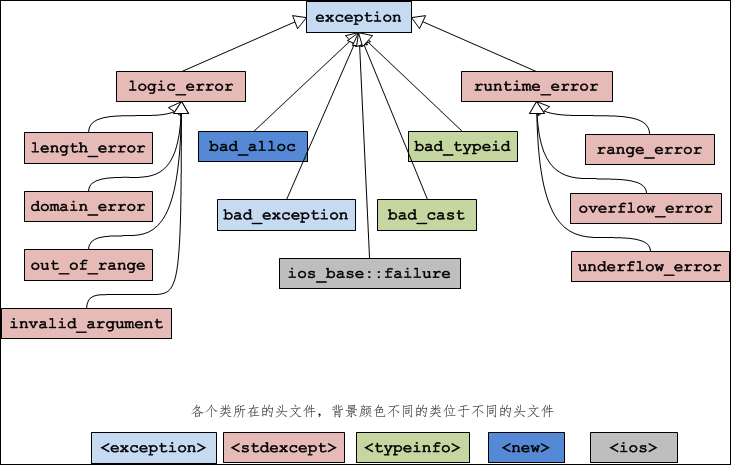std::exception该异常是所有标准 C++ 异常的父类。
std::logic_error理论上可以通过读取代码来检测到的异常。
std::domain_error当使用了一个无效的数学域时，会抛出该异常。
std::invalid_argument当使用了无效的参数时，会抛出该异常。
std::length_error当创建了太长的 std::string 时，会抛出该异常。
std::out_of_range该异常可以通过方法抛出，例如 std::vector 和 std::bitset<>::operator
std::runtime_error理论上不可以通过读取代码来检测到的异常。
std::overflow_error当发生数学上溢时，会抛出该异常。
std::range_error当尝试存储超出范围的值时，会抛出该异常。
std::underflow_error当发生数学下溢时，会抛出该异常。

# 21 STL

C++标准模板库（Standard Template Library,STL）是泛型程序设计最成功的实例。STL是一些常用数据结构和算法的模板的集合，由Alex Stepanov主持开发，于1998年被加入C++标准。

• C++ 标准模板库的核心包括三大组件：容器，算法，迭代器

### 21.1 容器

• 关联容器内的元素是排序的,所以查找时具有非常好的性能。
容器适配起：栈stack、队列queu、优先队列priority_queue
所有容器具有的函数：
int size();
bool empty();


begin()
end()
rbegin()
erase(...)
clear()


front()
back()
push_back();
pop_back();
insert(...);


### 21.2 迭代器

1.正向迭代器：容器类名::iterator 迭代器名;
2.常量正向迭代器：容器类名::const_iterator 迭代器名;
3.反向迭代器：容器类名::reverse_iterator 迭代器名;
4.常量反向迭代器：容器类名::const_reverse_iterator 迭代器名

### 21.3 算法

STL 提供能在各种容器中通用的算法（大约有70种），如插入、删除、查找、排序等。算法就是函数模板。算法通过迭代器来操纵容器中的元素。
STL 中的大部分常用算法都在头文件 algorithm 中定义。此外，头文件 numeric 中也有一些算法。
许多算法操作的是容器上的一个区间（也可以是整个容器），因此需要两个参数，一个是区间起点元素的迭代器，另一个是区间终点元素的后面一个元素的迭代器。

• copy：将一个容器的内容复制到另一个容器。
• remove：在容器中删除一个元素。
• random_shuffle：随机打乱容器中的元素。
• fill：用某个值填充容器。

• find：在容器中查找元素。
• count_if：统计容器中符合某种条件的元素的个数。
#include <vector>
#include <algorithm>
#include <iostream>
using namespace std;
int main()  {
vector<int> v;
v.push_back(1);
v.push_back(2);
v.push_back(3);
v.push_back(4); //1,2,3,4
vector<int>::iterator p;
p = find(v.begin(), v.end(), 3); //在v中查找3 若找不到,find返回 v.end()
if (p != v.end())
cout << "1) " << *p << endl; //找到了
p = find(v.begin(), v.end(), 9);
if (p == v.end())

p = find(v.begin() + 1, v.end() - 1, 4); //在2,3 这两个元素中查找4
cout << "2) " << *p << endl; //没找到，指向下一个元素4

int arr = { 10,20,30,40 };
int * pp = find(arr, arr + 4, 20);
if (pp == arr + 4)
else
cout << "3) " << *pp << endl;
return 0;

}
`

# 22 总结

终于写完了，因为新冠疫情，在家隔离了一个多月，闲来无事，写写博客来总结一下自己之前学的知识；零零散散大约花了一周左右的时间，该篇文章面向C++的基础知识，有许多详细，深层的没有写进去。后面有时间会写模板，STL，C++11新特性，Boost库等的文章，刚开始写博客，文章中有错误或有遗漏的知识点，请大佬们指出。03-141万+
10-243万+
12-242万+
08-093503
08-094387
04-25311
10-304300
01-2077
11-141203
04-196万+
03-21
03-021万+
11-17900
02-1688
08-26275
04-083852